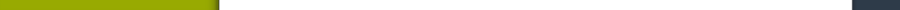Statistics course

The statistics

The ainm of this course is to introduce the basic principles of statistics for students.

The course focus mainly on introducing the basic concepts and basic methods of statistics to allow an autonomous learning of complementary methods.

We want to develop the critical necessary sense in the implementation and interpretation of statistical processing.

For this purpose, a rigorous mathematical framework will be introduced and used.